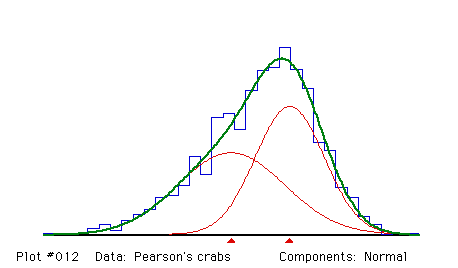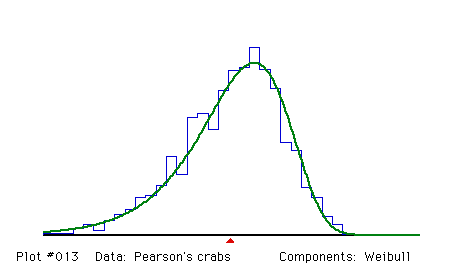## Karl Pearson's crab data

#### Source:

Pearson, K. (1894). Contributions to the mathematical theory of evolution. Phil. Trans. Roy. Soc. London A 185 , 71-110.

The data give the ratio of "forehead" breadth to body length for 1000 crabs sampled at Naples by Professor W.F.R. Weldon.

### Analysis 1:

The first analysis reproduces Pearson's original fit with two normal components.

#### Remarks:

Pearson (1894) analysed this histogram by the method of moments. The calculation was formidable and done without the aid of computing machinery of any kind. He found two solutions, one with 41.45% of the population in the first component and 58.55% in the second, the other with 53.28% in the first component and 46.72% in the second. He preferred the first solution on the basis of agreement with the sixth moment. MIX does not converge to a unique solution if all parameters are unconstrained. The iterations wander between a 6:4 and 4:6 ratio for the two components, with no fit being significantly better than any other. The standard errors of the proportions are quite large. The fit shown here resolves this uncertainty by constraining the proportions to be equal. The presence of two components was interpreted by Pearson as evidence that there were two species of crabs. I know of no biological justification for constraining the proportions to be equal, but the fit obtained is excellent. Constraining the standard deviations to be equal does not give an acceptable fit.``` Fitting Normal components

Proportions and their standard errors
.50000    .50000
FIXED     FIXED

Means and their standard errors
.6343     .6551
.0014     .0011

Sigmas and their standard errors
.0190     .0121
.0011     .0006

Degrees of freedom = 29 - 1 +   0 -  0 -  4 -   0 =  24

Chi-squared =  22.2055            (P =  .5670)```

### Analysis 2:

The data can also be fitted by a single negatively-skewed Weibull distribution.

#### Remarks:

Although not as good a fit as a mixture of two normals, a single Weibull component is an acceptable fit at the 1% level of significance. Since there was no independent biological evidence that the population was a mixture, the fact that a mixture of normals fits well does not prove that there are two species of crabs.``` Fitting Weibull components

Proportions and their standard errors
1.00000
FIXED

Means and their standard errors
.6443
.0006

Sigmas and their standard errors
.0207
.0005

Degrees of freedom = 29 - 1 +   0 -  0 -  2 -   0 =  26

Chi-squared =  44.9091            (P =  .0120)```
Back to the MIX Demonstration Examples Page